Measurement Units - Definition with Examples

The Complete K-5 Math Learning Program Built for Your Child

• 30 Million Kids

Loved by kids and parent worldwide

• 50,000 Schools

Trusted by teachers across schools

• Comprehensive Curriculum

Aligned to Common Core

Measurement Units

Measurement is to find a number that shows the amount of something. A measurement unit is a standard quantity used to express a physical quantity. Let us learn about the physical quantities and some of the standard units used to measure them.

Length

Length describes how long a thing is from one end to the other.

 Metric Unit US Standard Unit (English Unit or Customary Unit) Millimeter (mm) Used to measure very short lengths or thicknesses. Example, length of a pencil tip.   Centimeter (cm): Used to measure small lengths. Example: Length of a pencil.   Meter (m): Used to measure big lengths. Example: Length of a classroom.   Kilometer (km): Used to measure very long lengths or distance. Example: Distance between two places. Inch (in): Used to measure the length of small objects. Example: Length of a bed.   Foot (ft): Used to measure short distances and heights. Example: Heights of buildings.   Yard (yd): It is bigger than a foot. Example: Length of a football field.   Mile (mi): Used to measure long distances. Example: Distance between two places.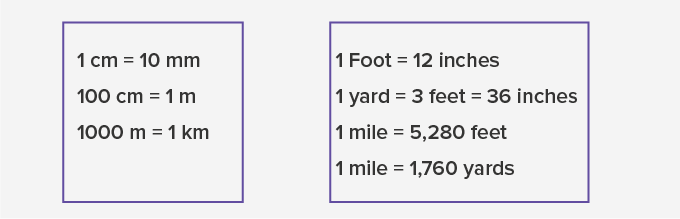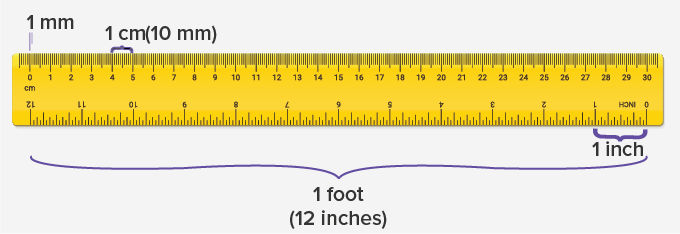Weight

The amount of matter a thing consists of is its weight.

 Metric Unit US Standard Unit (English Unit or Customary Unit) Milligram (mg): Used to measure very light things. Example: Medicines   Gram (g):  Used to measure small things. Example: Potato   Kilogram (kg):  Used to measure heavy things. Example: Body weight Ounce (oz):  Used to measure small quantities. Example: Bread.   Pound (lb):  Used to measure body weight, etc.   Ton:  Used to measure much heavier things. Example: Trucks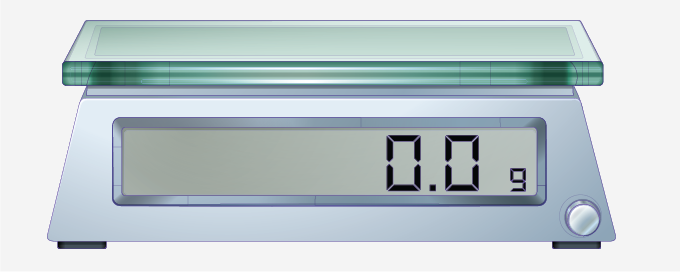Capacity (Volume)

Capacity is how much quantity a container can hold.

 Metric Unit US Standard Unit (English Unit or Customary Unit) Milliliter (ml): Used to measure very small quantities. Example: Medicines.   Liter (l):  Used to measure liquids. Example: juice, milk Cup  Pint Quart Gallon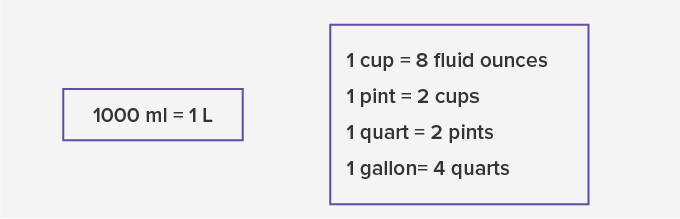Time

The ongoing sequence of events is time.

 Metric Units and US Standard Unit are the same for the time Second (s) Minute (min) Hour (hr) Day Week Month Year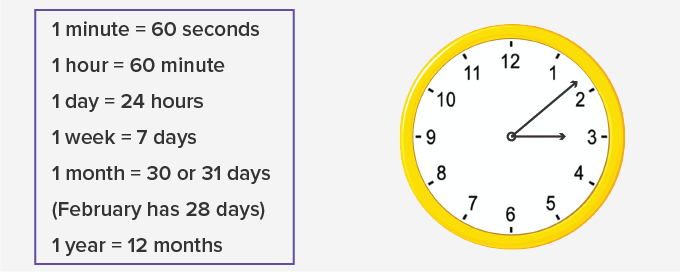Fun Fact Long before the standard units of measurement were made, non-standard units such as a digit, handspan, and pace were used.

Won Numerous Awards & Honors